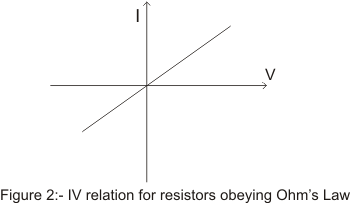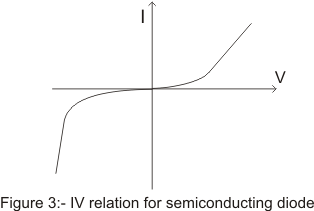# Variation of resistivity with temperature and current voltage relation

## (7) variation of resistivity with temperature

• Resistance and hence resistivity of conductor depends on mubers of factors
• One of the most important factors is dependence of resistance of metals on temperature
• Resistivity of the metallic conductor increases with increase on temperature
• when we increase the temperature of the metallic conductor,its constituent atoms vibrate with greater amplitudes then usual.This results
to the more frequent collison between ions and electrons
• As a result average time between the two successive collision decreases resulting the decrease in drift velocity
• Thus increase collison with the increase in tempearture results in increase resistivity
• For small temperature variations ,resistivity of the most of the metals varies according to the following relations
ρ(T)=ρ(T0)[1 + α(T-T0)]                    (14)
Where ρ(T) and ρ(T0) are the resistivies of the material at temperature T and T0 respectively and α is the constant for given materail and is known as coefficient of resistivity.
• Since resistance of a given conductors depends on the length and crosssectional area of the conductor through the relation
R=ρl/A
Hence temperature variation of the resistance can be given as
R=R(T0)[1 + α(T-T0)]                    (15)
• Resistivity of alloys also increase with temperature but this increase is much small as compared to metals
• Resistivies of the non-metals decreases with increase in temperature .This is because at high temperature more electrons becomes avialable for conduction as they set themselves loose from atoms and hence temperature coefficient of resistivity is negative for non-metals
• A similar behavior occurs in case of semi-conductors .temperature coefficient of resistivity is negative for semi-conductors and its value is often large for a semi-conductor materials

## (8) Current Voltage relations

• We know that current through any electrical device such as resistors depends on potential defference between the terminals
• Devices obeying ohm's law follow a linear relationship between current following and potential applied where current is directly proportional to voltage applied .Graphical relation between V and I is shown below in figure• Graph for a resistor obeying ohm's law is a straight line through the origin having some finite slope
• There are many electrical devices that does not obey the ohm's law and current may depends on voltage in more complicated ways.Such devices are called non-ohmic devices for examples vaccum tubes,semiconductor diodes ,transistors etc
• Consider the case of a semi conductor junction diode which are used to convert alternating current to direct current and are used to perform variety of logic functions is a non=ohmic device
• Graphical voltage relation for a diode is shown below in the figure• Figure clearly shows a non linear depeence of current on voltage and diode clearly does not follow the ohm's w
• When a device does not follow obey ohm's law,it has non linear voltage -current relation and the quantity V/I is no longer a constant however ratio is still known as resistance which now varies with current
• In such cases we define a quantity dV/dI known as dynamic resistance which expresses the relation between smaal change in current and resulting change in voltage
• Thus for non-ohmic electrical devices resistance is not constant for different values of V and I